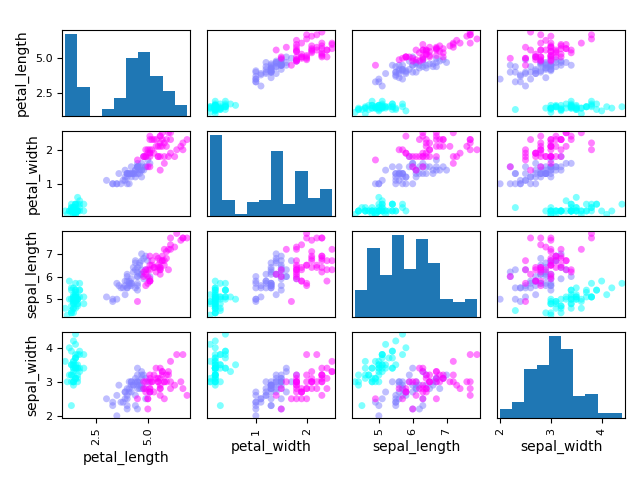# Scatter matrix¶

from __future__ import annotations

from gemseo.api import configure_logger
from gemseo.post.dataset.scatter_plot_matrix import ScatterMatrix

configure_logger()

<RootLogger root (INFO)>


iris = load_dataset("IrisDataset")


## Plot scatter matrix¶

We can use the ScatterMatrix plot where each non-diagonal block represents the samples according to the x- and y- coordinates names while the diagonal ones approximate the probability distributions of the variables, using either an histogram or a kernel-density estimator.

ScatterMatrix(iris, classifier="specy").execute(save=False, show=True)/home/docs/checkouts/readthedocs.org/user_builds/gemseo/envs/stable/lib/python3.9/site-packages/gemseo/post/dataset/scatter_plot_matrix.py:135: UserWarning: To output multiple subplots, the figure containing the passed axes is being cleared.
sub_axes = scatter_matrix(

[<Figure size 640x480 with 16 Axes>]


Total running time of the script: ( 0 minutes 0.700 seconds)

Gallery generated by Sphinx-Gallery